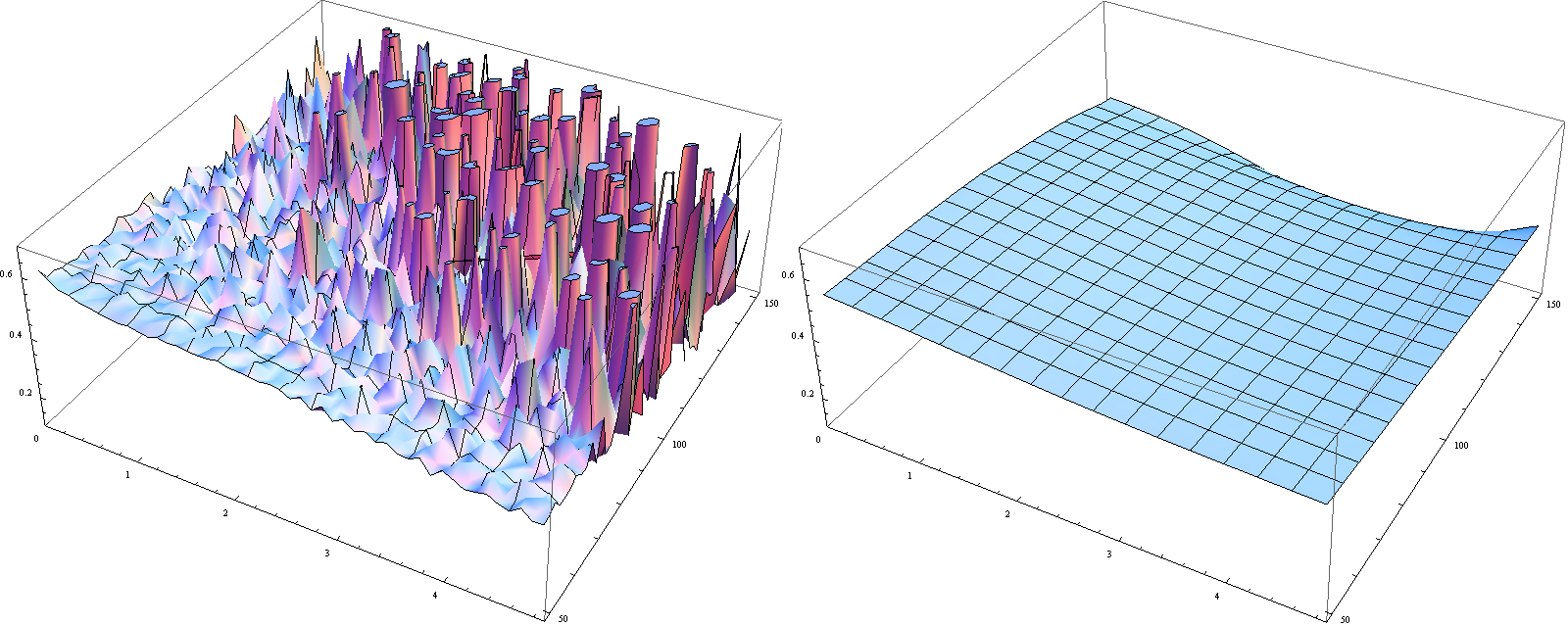## Pages

### Why are Adjoint Problems That Fast?

Last Monday, Hannes pointed out in his post on the Black Karasinski calibration that adjoint techniques are essential to obtain results in reasonable time.

For the sake of simplification, assume that we know the reversion speed and the volatility and we want to calibrate the drift parameter function theta. This problem is somehow similar to the Hull-White curve fitting problem (see also Austria is famous for skiing and calibration) but of higher complexity due to the lack of an analytic solution already for bond prices.

Remember that in both cases (Hull-White and Black-Karasinski), the drift terms are parameters in the respective partial differential equations (PDEs) that have to be solved for the valuation of financial instruments. For the Hull-White system, explicit solutions are available that need only the evaluation of a handful of integrals. In the Black-Karasinski case, the PDE has to be solved by numerical technques with finite elements being the preferred one.

In the calibration problem, the drift term has to be fitted to meet market bond prices (minimize an error functional plus some regularization term). This could either be done by calculate the so-called Gateaux derivatives in the directions of the changes of theta: This is the sensitivity approach which needs, for the calculation of the gradient, as many finite element solutions as there are design variables (i.e. free parameters in theta). The adjoint approach reformulates the problem and needs only one PDE solution for the gradient.Left: Naive application of Dupire formula, right: Egger-Engl utilizing regularization and adjoint techniques.